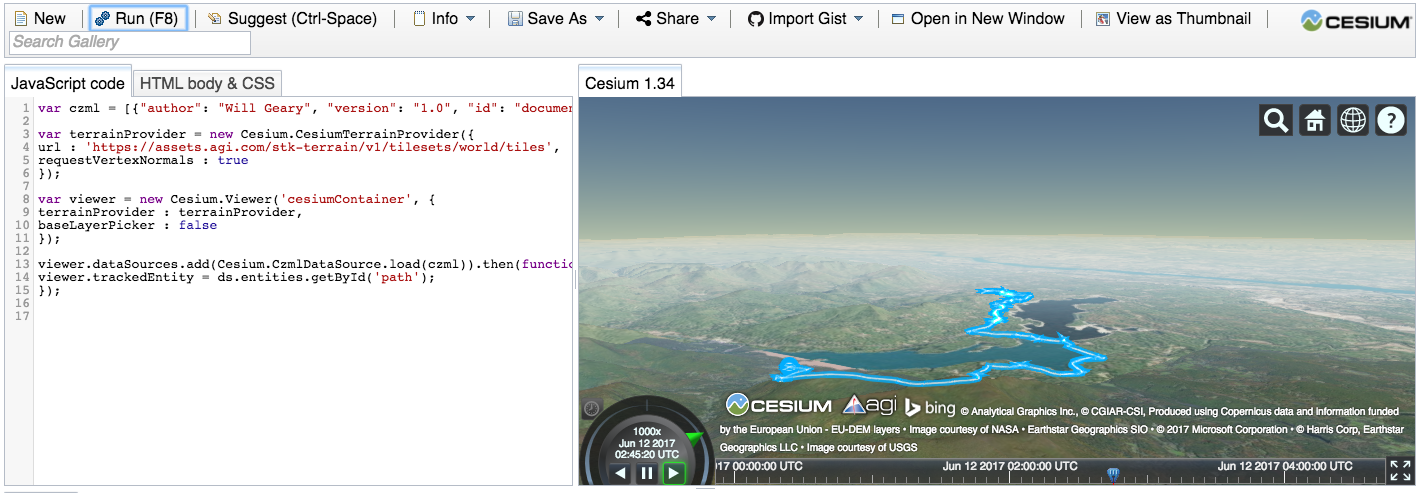# Visualizing a Bike Ride in 3D¶

Will Geary (@wgeary)

This notebook will walk through the steps necessary to use Cesium, a JavaScript library for 3D mapping, to visualize GPX data recorded with Strava.

Below is what the result looks like. Live version here: https://willgeary.github.io/CesiumApps/Apps/Strava/

In :
import IPython
url = 'https://willgeary.github.io/CesiumApps/Apps/Strava/'
iframe = '<iframe src=' + url + ' width=100% height=600></iframe>'
IPython.display.HTML(iframe)

Out:

Cesium defines a JSON data format called CZML for describing a time-dynamic graphical scenes, primarily for display in a web browser running Cesium. It describes lines, points, polygons, models, and other graphical primitives, and specifies how they change with time. The relationship between Cesium and CZML is similar to the relationship between Google Earth and KML.

CZML is an attractive paradigm for descriping spatio-temporal data. In particular, it proposes the following format for describing three-dimensional positions that change over time:

CartographicValue: A geodetic, WGS84 position specified as [Longitude, Latitude, Height]. If the array has three elements, the value is constant. If it has four or more elements, they are time-tagged samples arranged as [Time, Longitude, Latitude, Height, Time, Longitude, Latitude, Height, ...], where Time is an ISO 8601 date and time string or seconds since epoch.

For example, the following array specifies the [Long, Lat, Height] of an entity at 0 seconds since epoch, 1 second since epoch and 2 seconds since epoch:

[ 0, -77, 37, 100000,

1, -77.5, 37, 100500,

2, -77.9, 37, 100900 ]

# GPX + CZML = 3D Awesomeness¶

This notebook will walk through the following steps:

1. Read and parse GPX data
2. Use GPX data to generate CZML data
3. Visualize CZML data with CesiumJS

# Import Libraries¶

In :
import pandas as pd
import numpy as np
import gpxpy
import json


I used Strava to record a recent bike ride around the Ashokan Reservoir near Woodstock, NY. We will visualize the GPX file that was exported from Strava.

In :
gpx_file = open('../data/Woodstock_NY.gpx', 'r')
gpx = gpxpy.parse(gpx_file)


# Parse GPX File¶

Extract latitudes, longitudes, elevations and timestamps from GPX file and throw it into a dataframe.

In :
## See a primer on reading GPX data in python here: http://andykee.com/visualizing-strava-tracks-with-python.html

def parse_gpx(gpx_input_file):

lats = []
lons = []
elevations = []
timestamps = []

for track in gpx.tracks:
for segment in track.segments:
for point in segment.points:
lats.append(point.latitude)
lons.append(point.longitude)
elevations.append(point.elevation)
timestamps.append(point.time)

output = pd.DataFrame()
output['latitude'] = lats
output['longitude'] = lons
output['elevation'] = elevations
output['starttime'] = timestamps
output['stoptime'] = output['starttime'].shift(-1).fillna(method='ffill')
output['duration'] = (output['stoptime'] - output['starttime']) / np.timedelta64(1, 's') ## duration to seconds

return output


Here's what the GPX data looks like after we parse it:

In :
df = parse_gpx(gpx)

Out:
latitude longitude elevation starttime stoptime duration
0 42.029884 -74.107225 162.0 2017-06-11 19:51:59 2017-06-11 19:52:03 4.0
1 42.029855 -74.107269 163.0 2017-06-11 19:52:03 2017-06-11 19:52:05 2.0
2 42.029824 -74.107292 163.4 2017-06-11 19:52:05 2017-06-11 19:52:12 7.0
3 42.029788 -74.107308 163.4 2017-06-11 19:52:12 2017-06-11 19:52:20 8.0
4 42.029826 -74.107312 163.4 2017-06-11 19:52:20 2017-06-11 19:52:22 2.0

# Generate CZML Path¶

Create an array to describe three-dimensional position over time: [timestep, long, lat, elevation, ...]

In :
def create_czml_path(df_input, relative_elevation = False):
results = []

timestep = 0

for i in df_input.index:
results.append(timestep)
results.append(df_input.longitude.ix[i])
results.append(df_input.latitude.ix[i])

if relative_elevation == True:
results.append(30) # for use with point = {"heightReference" : "RELATIVE_TO_GROUND"}
else:
results.append(df_input.elevation.ix[i])

duration = df_input.duration.ix[(i)]
timestep += duration

return results


Here is what the first few positions look like on our path:

In :
create_czml_path(df)[:12]

Out:
[0,
-74.107225,
42.029884000000003,
162.0,
4.0,
-74.107269000000002,
42.029854999999998,
163.0,
6.0,
-74.107292000000001,
42.029823999999998,
163.40000000000001]

# Generate CZML Document¶

Now we need to create the entire CZML document, which will include the CZML path from above. See documenation here.

In :
def point_with_trailing_path(df_input, time_multiplier = 1000):

# Store output in array
czml_output = []

# Define global variables
global_id = "document"
global_name = "Visualizing GPX Data from Strava"
global_version = "1.0"
global_author = "Will Geary"
global_starttime = str(min(df_input['starttime'])).replace(" ", "T").replace(".000", "Z")
global_stoptime = str(max(df_input['stoptime'])).replace(" ", "T").replace(".000", "Z")
global_availability = global_starttime + "/" + global_stoptime

# Create packet with global variables
global_element = {
"id" : global_id,
"name" : global_name,
"version" : global_version,
"author": global_author,
"clock": {
"interval": global_availability,
"currentTime": global_starttime,
"multiplier": time_multiplier
}
}

# Append global packet to output
czml_output.append(global_element)

# Define path variables
path_id = "path"
path_starttime = str(min(df_input['starttime'])).replace(" ", "T").replace(".000", "Z")
path_stoptime = str(max(df_input['starttime'])).replace(" ", "T").replace(".000", "Z")
path_availability = path_starttime + "/" + path_stoptime

# Create path object
path_object = {
"id": path_id,

"availability": path_availability,

"position": {
"epoch": path_starttime,
"cartographicDegrees": create_czml_path(df, relative_elevation=False)
},

"path" : {
"material" : {
"polylineOutline" : {
"color" : {
"rgba" : [255,255,255, 200]
},
"outlineColor" : {
"rgba" : [0,173,253, 200]
},
"outlineWidth" : 5
}
},
"width" : 6,
"trailTime" : 100000,
"resolution" : 5
}
}

# Append path element to output
czml_output.append(path_object)

# Define point variable
point_id = "Point"
point_starttime = str(min(df_input['starttime'])).replace(" ", "T").replace(".000", "Z")
point_stoptime = str(max(df_input['starttime'])).replace(" ", "T").replace(".000", "Z")
point_availability = point_starttime + "/" + point_stoptime

point_object = {
"id": point_id,

"availability": point_availability,

"position": {
"epoch": point_starttime,
"cartographicDegrees": create_czml_path(df, relative_elevation=True)
},

"point": {
"color": {
"rgba": [255, 255, 255, 255]
},
"outlineColor": {
"rgba": [0,173,253, 255]
},
"outlineWidth":6,
"pixelSize":8,
"heightReference" : "RELATIVE_TO_GROUND"
}
}

czml_output.append(point_object)

return czml_output


Save output to czml file.

In :
czml_output = point_with_trailing_path(df)

with open('/Users/Will/node_modules/Cesium-1.33/Apps/Data/woodstock.czml', 'w') as outfile:
json.dump(czml_output, outfile)


# Visualize with Cesium¶

Below is what my result looks like (allow a few seconds to load). Full screen version here: https://willgeary.github.io/CesiumApps/Apps/Strava/

In :
import IPython
url = 'https://willgeary.github.io/CesiumApps/Apps/Strava/'
iframe = '<iframe src=' + url + ' width=100% height=600></iframe>'
IPython.display.HTML(iframe)

Out:

I created the above visualization using CesiumJS locally. But you can test yours out online without having to download Cesium by using Cesium's Sandbox tool.

1. Open the CZML output file created above and copy its contents

2. Paste CZML contents into the sidebar in place of existing czml variable

3. Click Run

You should get something like this:

In :
from IPython.display import Image
Image('../images/screenshot.png')

Out: